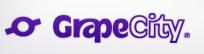# Placement Papers - GrapecityGRAPE CITY PLACEMENT PAPER (TECHNICALC, C++)
Posted by :
Sumit Sengupta
(20)
PAPER: GRAPE CITY PLACEMENT PAPER (TECHNICAL- C C++)

1.whenever the data type might be many bytes , & the function does not change the parameter within it body

2. A is a class & B is a new class derived from A

A a;

B b;

Bb1;

B b2;

3. what c++ syntax is used to declare that a class B is derived from Class A

a. class A derives B {};

b. class B: public A {,..};

4. using the variable , which is legat?

a. a=b;

b. b=a;

c. b1=b2;

d. both a & b are legal but not c;

e. both a & c are legal but not b;

f. both b & c are legal , but not a;

5. suppose there are 2 fns. F has an argument of type A and g has an argument of type B. Which is correct?

a. both f(a) & g(a) are legal fn. Calls

b. f(a) is legal , but g(a) is not legal

c. f(a) is not legal , g(a) is legal

d. neither f(a) nor g(a) is legal fn call

6. template

void foo(Item x);

which is right way to call with integer argument I?

a. foo(i);

b. foo (i);

c. foo(i);

d. foo( i);

e. foo( i);

7. void quiz(int w)

{

if(w>1)

{ quiz (w/2);

quiz(w/2);

}

printf("*);

}

how many asterisks are printed by the function call quiz(5)?

a. 3

b. 4

c. 7

d. 8

8. void test_a (int n)

{

printf("%d,n);

if(n>0)

test_a(n-2);

}

test_a(4)?

a . 0 2 4

c. 0 2

d. 2 4

e. 4 2

f. 4 2 0

9. char string=abcdefg;

*string=\'\';

printf("%s,string);

a. compiler error

b. run-time error

c. no o/p, but no error

d. creates bcdefg

10. char string=abcdefg

o/p :

printf("%s
,string +3);

abcdefg

abc

defg

cdefg

11. main()

{ int I=-3, j=2,k=0,m;

m=++I&&++j||++k;

printf("
%d%d%D, I,j,k,m);

A. "2 3 0 1

B. "2 3 1 1

C. "2 3 1 0

D. "2 3 0 0

12. main()

{

int I;

for(;;)

{

printf("%d,I++)

if(I>10)

break;

}

}

a. condition in a for-loop is mudt

b. no error

c. 2 ; shud be dropped

13.void goop ( int z[]);//prototype

int x;

which ois the correct way to call goop

a. goop(x);

b. goop(x[]);

c. goop(x);

d. goop(&x);

e. goop(&x[]);

14. int a=3,b=17;

a=b%a;

b=++a+5;

printf("a,b);

A. 2 8

B. 2 7

C. 3 7

D. 2 8

E. none

15. how many time hello will be printed?

FILE *fp=fopen("test.txt,w)

Fprintf(fp,hello);

Fork();

A. 1

B. 2

C. 0

D. none

16. int a;

int b=0;

while(a)

{

{ a&=a-1;

b++;

}

A. a &b

B. 0 & 15

C. 1 & 16

D. 0 & 16

E. none

17. class A

{

public:

static int a;

A() {a=10};

};

int main()

{

A b;

Printf("%d,b.a);

Return 0;

}

will the program compile?

a yes

b. no